# 10.8 Vectors  (Page 7/22)

 Page 7 / 22

## Verbal

What are the characteristics of the letters that are commonly used to represent vectors?

lowercase, bold letter, usually $\text{\hspace{0.17em}}u,v,w$

How is a vector more specific than a line segment?

What are $\text{\hspace{0.17em}}i\text{\hspace{0.17em}}$ and $\text{\hspace{0.17em}}j,$ and what do they represent?

They are unit vectors. They are used to represent the horizontal and vertical components of a vector. They each have a magnitude of 1.

What is component form?

When a unit vector is expressed as $⟨a,b⟩,$ which letter is the coefficient of the $\text{\hspace{0.17em}}i\text{\hspace{0.17em}}$ and which the $\text{\hspace{0.17em}}j?$

The first number always represents the coefficient of the $\text{\hspace{0.17em}}i,\text{\hspace{0.17em}}$ and the second represents the $\text{\hspace{0.17em}}j.$

## Algebraic

Given a vector with initial point $\text{\hspace{0.17em}}\left(5,2\right)\text{\hspace{0.17em}}$ and terminal point $\text{\hspace{0.17em}}\left(-1,-3\right),\text{\hspace{0.17em}}$ find an equivalent vector whose initial point is $\text{\hspace{0.17em}}\left(0,0\right).\text{\hspace{0.17em}}$ Write the vector in component form $⟨a,b⟩.$

Given a vector with initial point $\text{\hspace{0.17em}}\left(-4,2\right)\text{\hspace{0.17em}}$ and terminal point $\text{\hspace{0.17em}}\left(3,-3\right),\text{\hspace{0.17em}}$ find an equivalent vector whose initial point is $\text{\hspace{0.17em}}\left(0,0\right).\text{\hspace{0.17em}}$ Write the vector in component form $⟨a,b⟩.$

$〈7,-5〉$

Given a vector with initial point $\text{\hspace{0.17em}}\left(7,-1\right)\text{\hspace{0.17em}}$ and terminal point $\text{\hspace{0.17em}}\left(-1,-7\right),\text{\hspace{0.17em}}$ find an equivalent vector whose initial point is $\text{\hspace{0.17em}}\left(0,0\right).\text{\hspace{0.17em}}$ Write the vector in component form $⟨a,b⟩.$

For the following exercises, determine whether the two vectors $\text{\hspace{0.17em}}u\text{\hspace{0.17em}}$ and $\text{\hspace{0.17em}}v\text{\hspace{0.17em}}$ are equal, where $\text{\hspace{0.17em}}u\text{\hspace{0.17em}}$ has an initial point $\text{\hspace{0.17em}}{P}_{1}\text{\hspace{0.17em}}$ and a terminal point $\text{\hspace{0.17em}}{P}_{2}\text{\hspace{0.17em}}$ and $v$ has an initial point $\text{\hspace{0.17em}}{P}_{3}\text{\hspace{0.17em}}$ and a terminal point $\text{\hspace{0.17em}}{P}_{4}$ .

${P}_{1}=\left(5,1\right),{P}_{2}=\left(3,-2\right),{P}_{3}=\left(-1,3\right),\text{\hspace{0.17em}}$ and $\text{\hspace{0.17em}}{P}_{4}=\left(9,-4\right)$

not equal

${P}_{1}=\left(2,-3\right),{P}_{2}=\left(5,1\right),{P}_{3}=\left(6,-1\right),\text{\hspace{0.17em}}$ and $\text{\hspace{0.17em}}{P}_{4}=\left(9,3\right)$

${P}_{1}=\left(-1,-1\right),{P}_{2}=\left(-4,5\right),{P}_{3}=\left(-10,6\right),\text{\hspace{0.17em}}$ and $\text{\hspace{0.17em}}{P}_{4}=\left(-13,12\right)$

equal

${P}_{1}=\left(3,7\right),{P}_{2}=\left(2,1\right),{P}_{3}=\left(1,2\right),\text{\hspace{0.17em}}$ and $\text{\hspace{0.17em}}{P}_{4}=\left(-1,-4\right)$

${P}_{1}=\left(8,3\right),{P}_{2}=\left(6,5\right),{P}_{3}=\left(11,8\right),\text{\hspace{0.17em}}$ and ${P}_{4}=\left(9,10\right)$

equal

Given initial point $\text{\hspace{0.17em}}{P}_{1}=\left(-3,1\right)\text{\hspace{0.17em}}$ and terminal point $\text{\hspace{0.17em}}{P}_{2}=\left(5,2\right),\text{\hspace{0.17em}}$ write the vector $\text{\hspace{0.17em}}v\text{\hspace{0.17em}}$ in terms of $\text{\hspace{0.17em}}i\text{\hspace{0.17em}}$ and $\text{\hspace{0.17em}}j.\text{\hspace{0.17em}}$

Given initial point $\text{\hspace{0.17em}}{P}_{1}=\left(6,0\right)\text{\hspace{0.17em}}$ and terminal point $\text{\hspace{0.17em}}{P}_{2}=\left(-1,-3\right),\text{\hspace{0.17em}}$ write the vector $\text{\hspace{0.17em}}v\text{\hspace{0.17em}}$ in terms of $\text{\hspace{0.17em}}i\text{\hspace{0.17em}}$ and $\text{\hspace{0.17em}}j.\text{\hspace{0.17em}}$

$7i-3j$

For the following exercises, use the vectors u = i + 5 j , v = −2 i − 3 j ,  and w = 4 i j .

Find u + ( v w )

Find 4 v + 2 u

$-6i-2j$

For the following exercises, use the given vectors to compute u + v , u v , and 2 u − 3 v .

$u=⟨2,-3⟩,v=⟨1,5⟩$

$u=⟨-3,4⟩,v=⟨-2,1⟩$

$u+v=〈-5,5〉,u-v=〈-1,3〉,2u-3v=〈0,5〉$

Let v = −4 i + 3 j . Find a vector that is half the length and points in the same direction as $\text{\hspace{0.17em}}v.$

Let v = 5 i + 2 j . Find a vector that is twice the length and points in the opposite direction as $\text{\hspace{0.17em}}v.$

$-10i–4j$

For the following exercises, find a unit vector in the same direction as the given vector.

a = 3 i + 4 j

b = −2 i + 5 j

$-\frac{2\sqrt{29}}{29}i+\frac{5\sqrt{29}}{29}j$

c = 10 i j

$d=-\frac{1}{3}i+\frac{5}{2}j$

$-\frac{2\sqrt{229}}{229}i+\frac{15\sqrt{229}}{229}j$

u = 100 i + 200 j

u = −14 i + 2 j

$-\frac{7\sqrt{2}}{10}i+\frac{\sqrt{2}}{10}j$

For the following exercises, find the magnitude and direction of the vector, $\text{\hspace{0.17em}}0\le \theta <2\pi .$

$⟨0,4⟩$

$⟨6,5⟩$

$|v|=7.810,\theta =39.806°$

$⟨2,-5⟩$

$⟨-4,-6⟩$

$|v|=7.211,\theta =236.310°$

Given u = 3 i − 4 j and v = −2 i + 3 j , calculate $\text{\hspace{0.17em}}u\cdot v.$

Given u = − i j and v = i + 5 j , calculate $\text{\hspace{0.17em}}u\cdot v.$

$-6$

Given $\text{\hspace{0.17em}}u=⟨-2,4⟩\text{\hspace{0.17em}}$ and $\text{\hspace{0.17em}}v=⟨-3,1⟩,\text{\hspace{0.17em}}$ calculate $\text{\hspace{0.17em}}u\cdot v.$

Given u $=⟨-1,6⟩$ and v $=⟨6,-1⟩,$ calculate $\text{\hspace{0.17em}}u\cdot v.$

$-12$

## Graphical

For the following exercises, given $\text{\hspace{0.17em}}v,\text{\hspace{0.17em}}$ draw $v,$ 3 v and $\text{\hspace{0.17em}}\frac{1}{2}v.$

$⟨2,-1⟩$

$⟨-1,4⟩$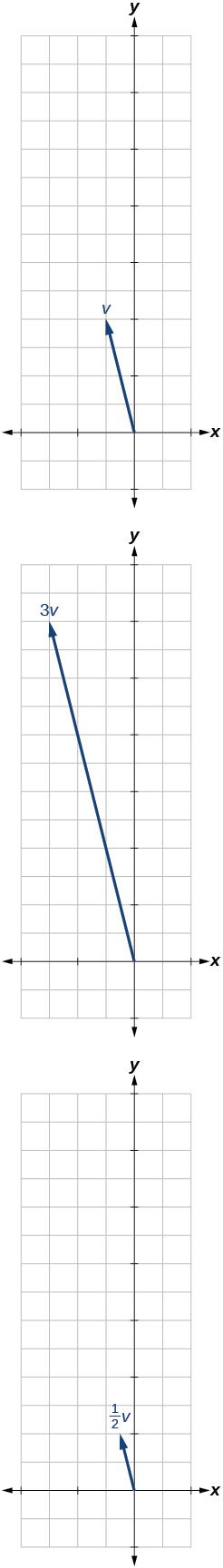$⟨-3,-2⟩$

For the following exercises, use the vectors shown to sketch u + v , u v , and 2 u .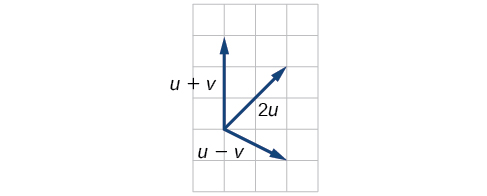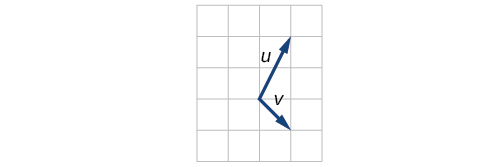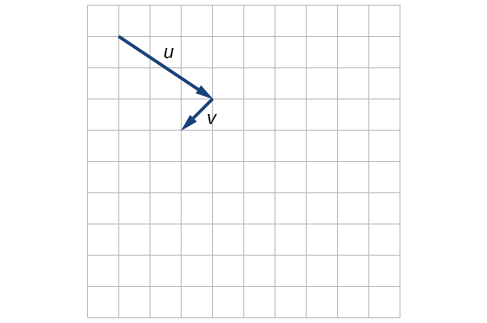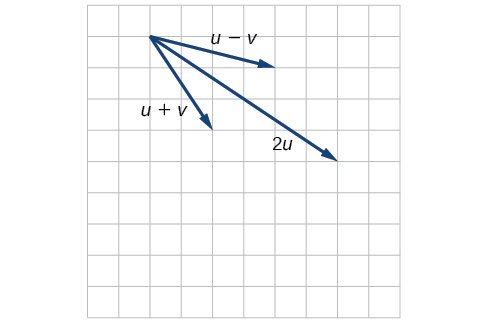For the following exercises, use the vectors shown to sketch 2 u + v .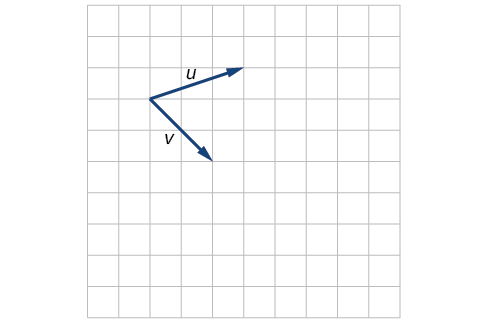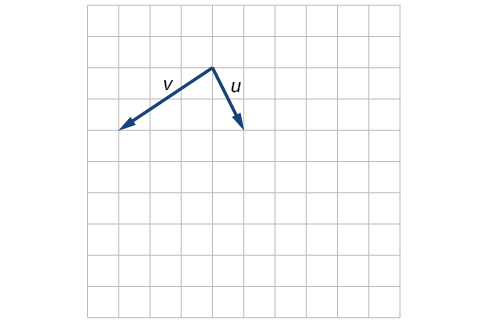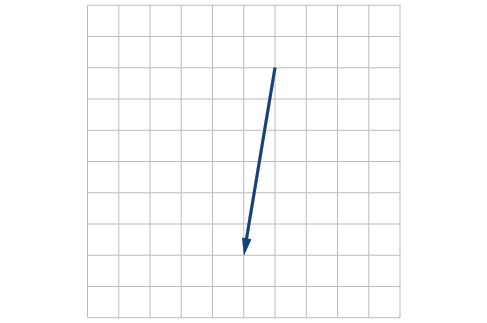For the following exercises, use the vectors shown to sketch u − 3 v .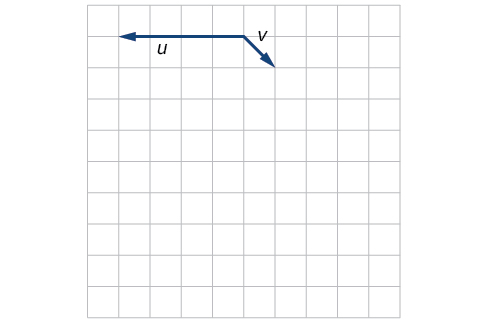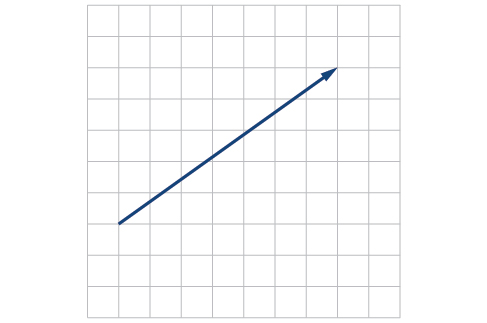For the following exercises, write the vector shown in component form.

#### Questions & Answers

The sequence is {1,-1,1-1.....} has
amit Reply
circular region of radious
Kainat Reply
how can we solve this problem
Joel Reply
Sin(A+B) = sinBcosA+cosBsinA
Eseka Reply
Prove it
Eseka
Please prove it
Eseka
hi
Joel
June needs 45 gallons of punch. 2 different coolers. Bigger cooler is 5 times as large as smaller cooler. How many gallons in each cooler?
Arleathia Reply
find the sum of 28th term of the AP 3+10+17+---------
Prince Reply
I think you should say "28 terms" instead of "28th term"
Vedant
if sequence sn is a such that sn>0 for all n and lim sn=0than prove that lim (s1 s2............ sn) ke hole power n =n
SANDESH Reply
write down the polynomial function with root 1/3,2,-3 with solution
Gift Reply
if A and B are subspaces of V prove that (A+B)/B=A/(A-B)
Pream Reply
write down the value of each of the following in surd form a)cos(-65°) b)sin(-180°)c)tan(225°)d)tan(135°)
Oroke Reply
Prove that (sinA/1-cosA - 1-cosA/sinA) (cosA/1-sinA - 1-sinA/cosA) = 4
kiruba Reply
what is the answer to dividing negative index
Morosi Reply
In a triangle ABC prove that. (b+c)cosA+(c+a)cosB+(a+b)cisC=a+b+c.
Shivam Reply
give me the waec 2019 questions
Aaron Reply
the polar co-ordinate of the point (-1, -1)
Sumit Reply

### Read also:

#### Get the best Algebra and trigonometry course in your pocket!

Source:  OpenStax, Algebra and trigonometry. OpenStax CNX. Nov 14, 2016 Download for free at https://legacy.cnx.org/content/col11758/1.6
Google Play and the Google Play logo are trademarks of Google Inc.

Notification Switch

Would you like to follow the 'Algebra and trigonometry' conversation and receive update notifications?ByByBy